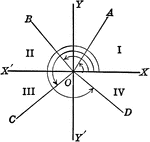Axes showing trigonometric angles, and quadrants.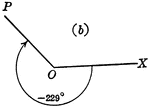### -229 Degree Trigonometric Angle

Trigonometric angle -229 degrees.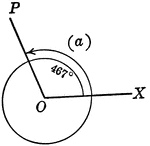### 467 Degree Trigonometric Angle

Trigonometric angle 467 degrees.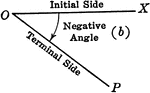### Negative Trigonometric Angle

Negative trigonometric angle with initial and terminal sides labeled.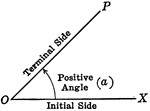### Positive Trigonometric Angle

Positive trigonometric angle with initial and terminal sides labeled.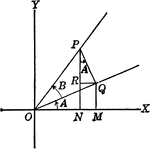### Angles Used to Illustrate Sum and Difference of Two Angles

Angles used to illustrate the sum and difference of two angles and trig identities.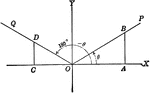### Coordinate Axis With Angles, Lines, and Perpendiculars Drawn

Coordinate axis with angle XOP equal to theta, Θ, and angle XOQ=180 - Θ. From any point in the terminal…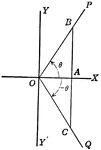### Coordinate Axis With Perpendiculars Drawn To Form Similar Right Triangles From Positive and Negative Theta, Θ

Angle XOP=Θ and angle XOQ=- Θ. From a point in the terminal side of each a perpendicular line is drawn…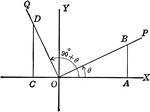### Coordinate Axis With Perpendiculars Drawn To Form Similar Right Triangles

Angle XOP=Θ and angle XOQ=90+Θ. From a point in the terminal side of each a perpendicular line is…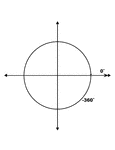### 0° and -360° Coterminal Angles

Illustration showing coterminal angles of 0° and -360°. Coterminal angles are angles drawn in…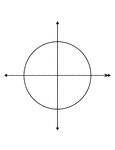### 0° and -360° Coterminal Angles

Illustration showing coterminal angles of 0° and -360°. Coterminal angles are angles drawn in…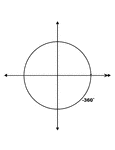### 0° and -360° Coterminal Angles

Illustration showing coterminal angles of 0° and -360°. Coterminal angles are angles drawn in…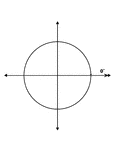### 0° and -360° Coterminal Angles

Illustration showing coterminal angles of 0° and -360°. Coterminal angles are angles drawn in…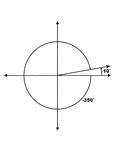### 10° and -350° Coterminal Angles

Illustration showing coterminal angles of 10° and -350°. Coterminal angles are angles drawn…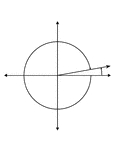### 10° and -350° Coterminal Angles

Illustration showing coterminal angles of 10° and -350°. Coterminal angles are angles drawn…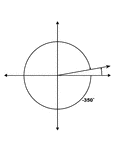### 10° and -350° Coterminal Angles

Illustration showing coterminal angles of 10° and -350°. Coterminal angles are angles drawn…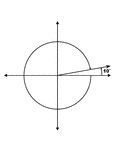### 10° and -350° Coterminal Angles

Illustration showing coterminal angles of 10° and -350°. Coterminal angles are angles drawn…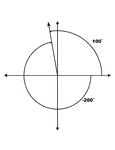### 100° and -260° Coterminal Angles

Illustration showing coterminal angles of 100° and -260°. Coterminal angles are angles drawn…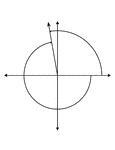### 100° and -260° Coterminal Angles

Illustration showing coterminal angles of 100° and -260°. Coterminal angles are angles drawn…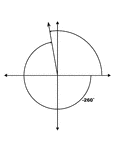### 100° and -260° Coterminal Angles

Illustration showing coterminal angles of 100° and -260°. Coterminal angles are angles drawn…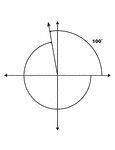### 100° and -260° Coterminal Angles

Illustration showing coterminal angles of 100° and -260°. Coterminal angles are angles drawn…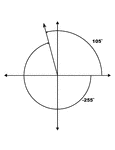### 105° and -255° Coterminal Angles

Illustration showing coterminal angles of 105° and -255°. Coterminal angles are angles drawn…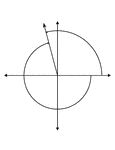### 105° and -255° Coterminal Angles

Illustration showing coterminal angles of 105° and -255°. Coterminal angles are angles drawn…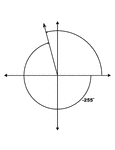### 105° and -255° Coterminal Angles

Illustration showing coterminal angles of 105° and -255°. Coterminal angles are angles drawn…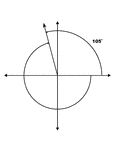### 105° and -255° Coterminal Angles

Illustration showing coterminal angles of 105° and -255°. Coterminal angles are angles drawn…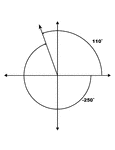### 110° and -250° Coterminal Angles

Illustration showing coterminal angles of 110° and -250°. Coterminal angles are angles drawn…### 110° and -250° Coterminal Angles

Illustration showing coterminal angles of 110° and -250°. Coterminal angles are angles drawn…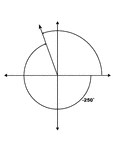### 110° and -250° Coterminal Angles

Illustration showing coterminal angles of 110° and -250°. Coterminal angles are angles drawn…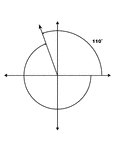### 110° and -250° Coterminal Angles

Illustration showing coterminal angles of 110° and -250°. Coterminal angles are angles drawn…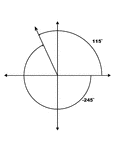### 115° and -245° Coterminal Angles

Illustration showing coterminal angles of 115° and -245°. Coterminal angles are angles drawn…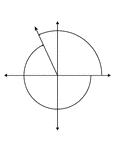### 115° and -245° Coterminal Angles

Illustration showing coterminal angles of 115° and -245°. Coterminal angles are angles drawn…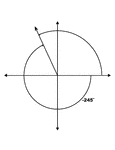### 115° and -245° Coterminal Angles

Illustration showing coterminal angles of 115° and -245°. Coterminal angles are angles drawn…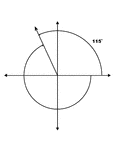### 115° and -245° Coterminal Angles

Illustration showing coterminal angles of 115° and -245°. Coterminal angles are angles drawn…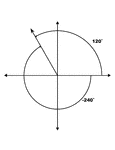### 120° and -240° Coterminal Angles

Illustration showing coterminal angles of 120° and -240°. Coterminal angles are angles drawn…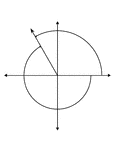### 120° and -240° Coterminal Angles

Illustration showing coterminal angles of 120° and -240°. Coterminal angles are angles drawn…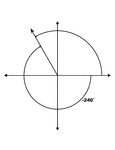### 120° and -240° Coterminal Angles

Illustration showing coterminal angles of 120° and -240°. Coterminal angles are angles drawn…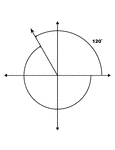### 120° and -240° Coterminal Angles

Illustration showing coterminal angles of 120° and -240°. Coterminal angles are angles drawn…### 125° and -235° Coterminal Angles

Illustration showing coterminal angles of 125° and -235°. Coterminal angles are angles drawn…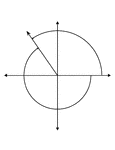### 125° and -235° Coterminal Angles

Illustration showing coterminal angles of 125° and -235°. Coterminal angles are angles drawn…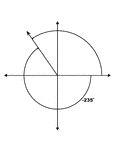### 125° and -235° Coterminal Angles

Illustration showing coterminal angles of 125° and -235°. Coterminal angles are angles drawn…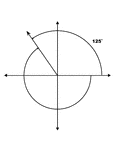### 125° and -235° Coterminal Angles

Illustration showing coterminal angles of 125° and -235°. Coterminal angles are angles drawn…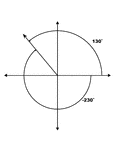### 130° and -230° Coterminal Angles

Illustration showing coterminal angles of 130° and -230°. Coterminal angles are angles drawn…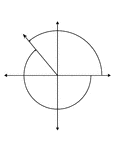### 130° and -230° Coterminal Angles

Illustration showing coterminal angles of 130° and -230°. Coterminal angles are angles drawn…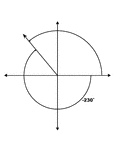### 130° and -230° Coterminal Angles

Illustration showing coterminal angles of 130° and -230°. Coterminal angles are angles drawn…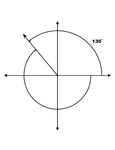### 130° and -230° Coterminal Angles

Illustration showing coterminal angles of 130° and -230°. Coterminal angles are angles drawn…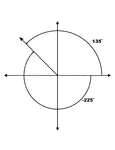### 135° and -225° Coterminal Angles

Illustration showing coterminal angles of 135° and -225°. Coterminal angles are angles drawn…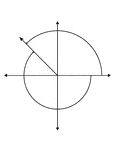### 135° and -225° Coterminal Angles

Illustration showing coterminal angles of 135° and -225°. Coterminal angles are angles drawn…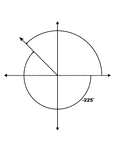### 135° and -225° Coterminal Angles

Illustration showing coterminal angles of 135° and -225°. Coterminal angles are angles drawn…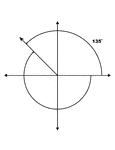### 135° and -225° Coterminal Angles

Illustration showing coterminal angles of 135° and -225°. Coterminal angles are angles drawn…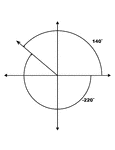### 140° and -220° Coterminal Angles

Illustration showing coterminal angles of 140° and -220°. Coterminal angles are angles drawn…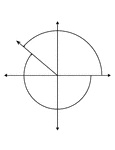### 140° and -220° Coterminal Angles

Illustration showing coterminal angles of 140° and -220°. Coterminal angles are angles drawn…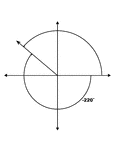### 140° and -220° Coterminal Angles

Illustration showing coterminal angles of 140° and -220°. Coterminal angles are angles drawn…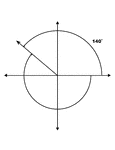### 140° and -220° Coterminal Angles

Illustration showing coterminal angles of 140° and -220°. Coterminal angles are angles drawn…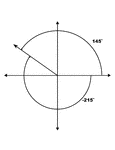### 145° and -215° Coterminal Angles

Illustration showing coterminal angles of 145° and -215°. Coterminal angles are angles drawn…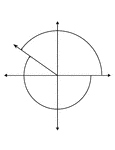### 145° and -215° Coterminal Angles

Illustration showing coterminal angles of 145° and -215°. Coterminal angles are angles drawn…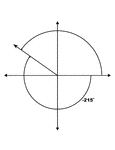### 145° and -215° Coterminal Angles

Illustration showing coterminal angles of 145° and -215°. Coterminal angles are angles drawn…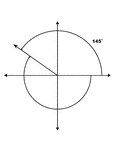### 145° and -215° Coterminal Angles

Illustration showing coterminal angles of 145° and -215°. Coterminal angles are angles drawn…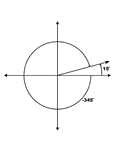### 15° and -345° Coterminal Angles

Illustration showing coterminal angles of 15° and -345°. Coterminal angles are angles drawn…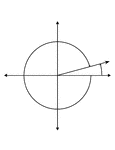### 15° and -345° Coterminal Angles

Illustration showing coterminal angles of 15° and -345°. Coterminal angles are angles drawn…### 15° and -345° Coterminal Angles

Illustration showing coterminal angles of 15° and -345°. Coterminal angles are angles drawn…